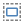# Form Field Calculations

PDF Studio is capable of creating PDF forms with custom calculations. You have the ability to use some of the preset simple calculations or advanced custom calculations using JavaScript. This is very useful for when you want values entered into a form calculated automatically. For example: summing up a total on an invoice sheet to give to a customer.

1. Start the Form Editing Mode by going to the Forms Tab
• Note: Make sure to create all of the Fields that you need in the document first. For more information regarding on how to create Fields see Creating Text Fields. Also, Make sure that you have correctly named all of the Form Fields in your document to make it easier to setup the calculations
2. Using the Object Selection Toolselect the Field that you would like to perform the calculation
3. Access the field properties by right clicking (Mac: Ctrl + Click) on the field and choose Properties in the mouse menu or click on the edit buttonon the Mini Toolbar.
4. In the Properties window select the Calculate tab
5. Use this screen to set any of the Form Field Calculation Options listed below. Once you have completed setting your calculations click on OK
6. Repeat steps 2- 5 to create as many fields with calculations as you need.
7. Once all calculations are complete click on the Stop Form Editing button on the toolbar to close the form editor and save the document
8. The Form Calculations will now be ready for you to use

### Form Field Calculation Options

#### No Calculation for this field

Default value, sets no calculation rules and any text can be entered into the field

#### Field value has simple calculation

When selected this option allows you to set a single math calculation on either all or a selection of fields. To Setup a simple calculation follow the steps below

1. Select the option for “Field value has a simple calculation:”
2. Then select the type of calculation you would like from the drop down menu. You have the below options available for simple calculations:
• Sum (+) – adds the fields together
• Product (x) – multiplies the fields together
• Average – returns the average value of all the fields
• Minimum – Returns the lowest value from all the fields
• Maximum – Returns the highest value of all the fields
3. Select the fields from the list you would like to be included in the calculation. To select more than one field from the list either click and drag the mouse or hold Ctrl and click the fields that you need from the list. Only highlighted fields will be used in the calculation.
• Note:  (You can also select other fields such as Combo or List Boxes to be used in calculations)

#### This field has a custom calculation

The Custom Calculation allows you to perform more advanced calculations using JavaSript. You will need to have advance knowledge in programming JavaScript in order to create the calculations. For more information regarding using JavaScript in PDF Studio see PDF Form JavaScript.

Select the option “This field has a custom calculation:” and then type in the JavaScript calculation that you would like to perform.

#### Simple Field Notation

Simple field notation allows you to use simple calculation commands (+ - / *) instead of JavaScript. For Example to add Text1 to Text 2 you would type "Text1 + Text2". Once complete PDF Studio will convert the simple annotation into JavaScript that is compatible with the PDF format.

1. Select the option “This field has a custom calculation:” and check the box for "Simple Field Notation"
2. Then use the custom calculation field to enter your calculations.

Note: When creating custom calculations the field name must exactly match the name set in the field properties in order for the calculation to work.

The following math functions are available:

• +: adds the two fields
• -: subtracts the two fields
• x: multiplies the two fields together
• /: divides the two fields
• (): groups the equations within the parenthesis as a separate function

Affordable, Powerful PDF Software / PDF Editor for Windows, macOS, Linux